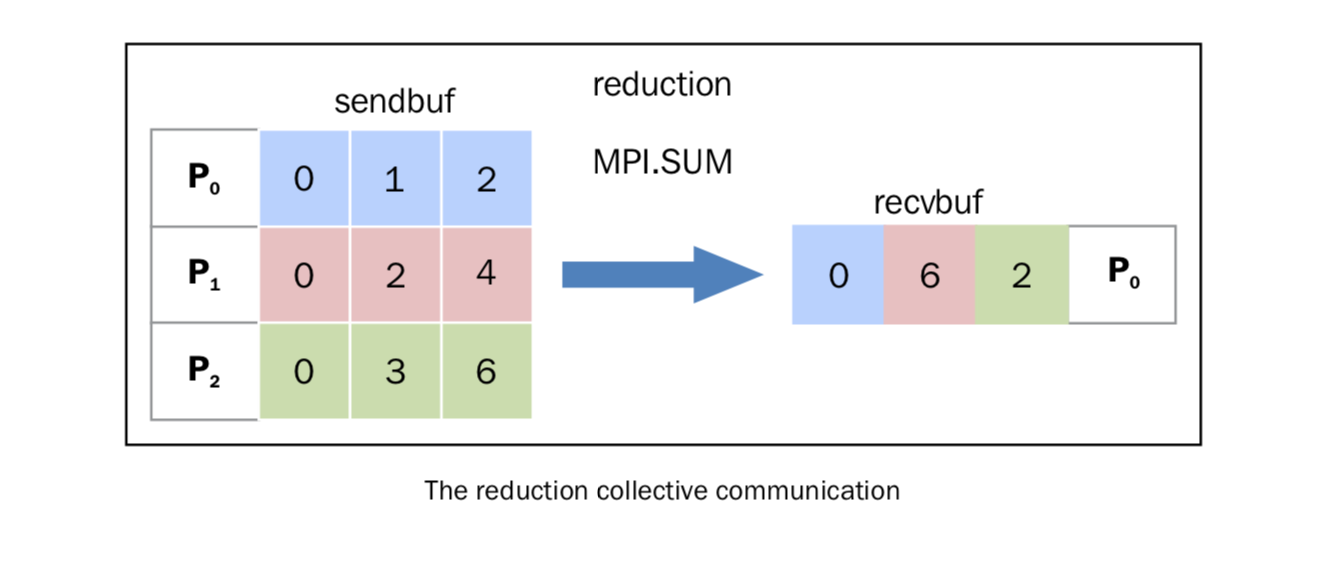# 18. 简化操作¶

`comm.gather` 一样， `comm.reduce` 接收一个数组，每一个元素是一个进程的输入，然后返回一个数组，每一个元素是进程的输出，返回给 root 进程。输出的元素中包含了简化的结果。

`mpi4py` 中，我们将简化操作定义如下：

```comm.Reduce(sendbuf, recvbuf, rank_of_root_process, op = type_of_reduction_operation)
```

• `MPI.MAX` : 返回最大的元素
• `MPI.MIN` : 返回最小的元素
• `MPI.SUM` : 对所有元素相加
• `MPI.PROD` : 对所有元素相乘
• `MPI.LAND` : 对所有元素进行逻辑操作
• `MPI.MAXLOC` : 返回最大值，以及拥有它的进程
• `MPI.MINLOC` : 返回最小值，以及拥有它的进程

## 18.1. 如何做…¶

```import numpy
import numpy as np
from mpi4py import MPI
comm = MPI.COMM_WORLD
size = comm.size
rank = comm.rank
array_size = 3
recvdata = numpy.zeros(array_size, dtype=numpy.int)
senddata = (rank+1)*numpy.arange(size,dtype=numpy.int)
print("process %s sending %s " % (rank , senddata))
comm.Reduce(senddata, recvdata, root=0, op=MPI.SUM)
print('on task', rank, 'after Reduce:    data = ', recvdata)
```

```C:\>mpiexec -n 3 python reduction2.py
process 2 sending [0 3 6]
on task 2 after Reduce:    data =  [0 0 0]
process 1 sending [0 2 4]
on task 1 after Reduce:    data =  [0 0 0]
process 0 sending [0 1 2]
on task 0 after Reduce:    data =  [ 0  6 12]
```

## 18.2. 讨论¶

```comm.Reduce(senddata, recvdata, root=0, op=MPI.SUM)
```• 进程 P0 发出数据数组 [0 1 2]
• 进程 P1 发出数据数组 [0 2 4]
• 进程 P2 发出数据数组 [0 3 6]

（译注：“简化”翻译的可能不太合适）